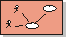# Guidelines: Requirements: – Use-Case DiagramUse-Case Diagram A use-case diagram shows actors, use cases, use-case packages, and their relationships.

Diagrams with actors, use cases, and relationships among them are called use-case diagrams and illustrate relationships in the use-case model.

Use-case diagrams can be organized into (and owned by) use-case packages, showing only what is relevant within a particular package.

### UseThere are no strict rules about what to illustrate in use-case diagrams. Show what you think are interesting relationships in the model. The following diagrams may be of interest:

• Actors belonging to the same use-case package.
• An actor and all the use cases with which it interacts. A diagram of this type can function as a local diagram of the actor, and is likely to be related to it.
• Use cases that handle the same information.
• Use cases used by the same group of actors.
• Use cases that are often executed in one sequence.
• Use cases that belong to the same use-case package.
• The most important use cases. A diagram of this type can function as a summary of the model, and is likely to be included in the use-case view.
• The use cases developed together (within the same increment).
• A specific use case and its relationships to actors and other use cases. A diagram of this type can function as a local diagram of the use case, and is likely to be related to it.

It is recommended that you include each actor, use case, and relationship in at least one of the diagrams. If it makes the use-case model clearer, they can be part of several diagrams and you can show them several times in the same diagram.